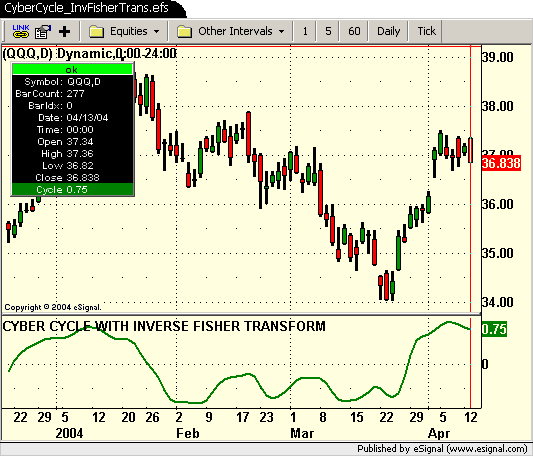# 2004 May: CyberCycle_InvFisherTrans.efs

ICE Data Services -

CyberCycle_InvFisherTrans.efs

File Name: CyberCycle_InvFisherTrans.efs

Description:
Based on The Inverse Fisher Transform by John Ehlers. This article appeared in the May 2004 issue of Stock & Commodities.

Formula Parameters:

• alpha - 0.07

Notes:
The related article is copyrighted material. If you are not a subscriber of Stocks & Commodities, please visit www.traders.com.
The May 2004 EFS formulas were provided by TS Support.
The current version was modified by eSignal for real time intraday analysis 4/20/2004.

CyberCycle_InvFisherTrans.efsEFS Code:

```// Magazine:	Technical Analysis of Stocks & Commodities, May 2004
// Article:		The Inverse Fisher Transform by John F. Ehlers
// Study:		CYBER CYCLE WITH INVERSE FISHER TRANSFORM
// Provided By:	TS Support, LLC for eSignal

// Version 1.1  4/20/2004       Modified by eSignal for real time analysis

var Smooth = 0;
var Smooth_1 = 0;
var Smooth_2 = 0;
var Cycle = 0;
var Cycle_1 = 0;
var Cycle_2 = 0;

function preMain(){
setStudyTitle("CYBER CYCLE WITH INVERSE FISHER TRANSFORM");
setCursorLabelName("Cycle",0);
setDefaultBarFgColor(Color.green,0);
setDefaultBarThickness(2);
//setComputeOnClose();
}

function main(alpha){
if(alpha == null) alpha = .07;
var ICycle = 0;

if (getBarState() == BARSTATE_NEWBAR) {
Smooth_2 = Smooth_1;
Smooth_1 = Smooth;
Cycle_2 = Cycle_1;
Cycle_1 = Cycle;
}

Smooth = ((high() + low()) / 2 + high(-1) + low(-1) + high(-2) + low(-2) + (high(-3) + low(-3)) / 2 ) / 6;
Cycle = (1 - .5 * alpha) * (1 - .5 * alpha) * (Smooth - 2 * Smooth_1 + Smooth_2) + 2 * (1 - alpha) * Cycle_1 - (1 - alpha) * (1 - alpha) * Cycle_2;
if(getCurrentBarIndex() - getOldestBarIndex() < 7)
Cycle = ((high() + low()) / 2 - high(-1) - low(-1) + high(-2) + low(-2)) / 4;
ICycle = (Math.exp(2 * Cycle) - 1) / (Math.exp(2 * Cycle) + 1);

return ICycle;
}```